# SSL Minutes

1 Feb 2001
Hal and Geir

## Jim Propp Talks about SSL.

Each individual is expected to create something (code, illustrations, documents, proofs).

Slogan: Teach what you know, learn what you don't, document everything.''

Grant Proposal.

Somebody is in charge of keeping the Glossary.

Disseminate everything on the web.

Shared Bonuses. For example, Prove the Baxter Permutation Conjecture for an hour's extra pay (for everybody).

Earn an extra hour's wages by attending Combinatorics Seminar 2:25-3:15 M 903 VV (next week only)

Get Time Cards. 2nd floor VV. Hand it to Propp or put it in his mailbox. Today counts as work.

We can spend money on supplies, for example, books. We have a LaTeX book somewhere.

Homework #1: Count the number of Domino tilings for 2xN rectangle, for n<=10. There is a pattern there. Find it and exploit it.

Public Web Site: http://jamespropp.org/SSL/ There is a private web site and an email list.

Accounts on dodgeson will be done on Thursday.

## Mathematics:

We know some things about an Aztec Diamond. But what about a rectangle or square?

The number of domino tilings of a 2n-by-2n square is:

\begin{displaymath} \product_{j=1}^n \product_{k=1}^n (4 \cos^2 \frac{\pi j}{2n+1} + 4 \cos^2 \frac{\pi k}{2n+1}) \end{displaymath}

Is it an integer? Yes: you can use Galois theory to show that it's a rational number and algebraic number theory to show that it's an "algebraic integer". The only numbers that are both rational and algebraic integers are integers.

To prove that something is an integer: (1) Cook up a combinatorial problem it describes (2) Reverse engineer the algebra.

A different kind of tiling problem.

Domino vs. Lozenge tilings: (Fig 1 and 2)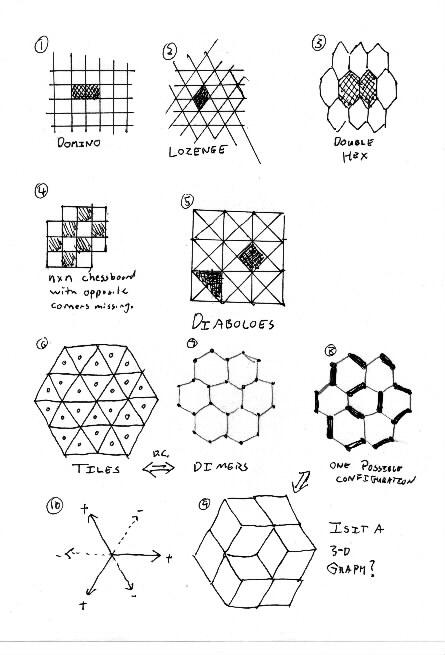Dominos are 1x2 rectangles in a grid. Lozenges are two touching equilateral triangles.

Double-Hexes (Fig 3) don't do anything cool, because they don't have height functions.

Not all areas are tilable: Hal asks about the nxn chessboard missing two opposite corners. (Fig 4)

Also look at Diaboloes; (fig 5) they are also interesting.

One can color tiles alternating colors if each vertex has an even number of cells touching it.

Not going to talk about dual graphs. The dual graph is bipartite.''

The Dimer Problem.

Look at the dual correspondence between tiles and Dimers in figures 6 and 7. In Fig 6, we need to connect triangles that share edges to form lozenges.

In Figure 7, we need to find a dimer configuration such that each vertex is paired with exactly one neighbor.

Pick a random configuration: notice that once you make a few decisions, others are forced upon you. (Fig 8)

Now convert it back to lozenge tilings. Suddenly, we see a 3-d cube figure! (Fig 9) Figure 10 shows positive and negative directions.

Now Boytcho produces a double die so that we can see a cube within a cube.

Let height be defined as h(x,y,z) = x + y + z

Figure 11 shows the height of each vertex.

Figure 1 shows Abe's 2-d analog, so we understand what height really means. In this figure, the line x+y=0 represents height=0. The point (1,2) has height=3. Its real'' distance from the h=0 line is 3/\sqrt{2}. But we like integers!

Now we need to mathematically define a way to calculate what your eye sees as height.

Height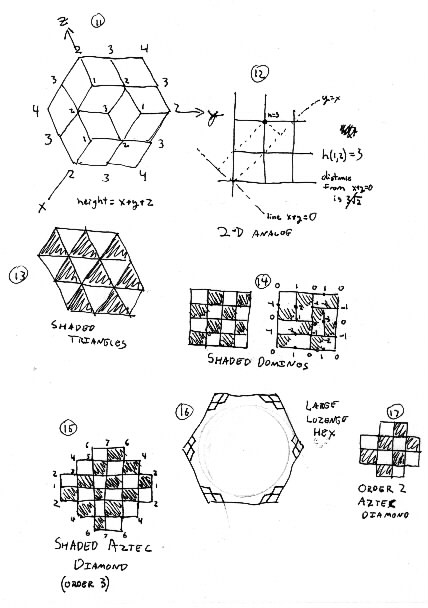This way we generalize what a height function is. We can define the height of a vertex on a domino graph by analogy to lozenges. (Fig 14)

On a square, the boundaries must be alternating zeros and ones on top and bottom, and alternating zeros and minus ones on left and right. Boring.

But on an Aztec Diamond of order 3, the height goes up from 1 to 7 as we go from left to top, then back down and up as we go around the other sides. (Fig 15) These different boundary conditions change how the inside behaves. The Aztec diamond is approximately a saddle shaped surface. That's why the corners freeze out.

You can think of a large lozenge hexagon as a monkey saddle. All six corners freeze out, leaving a circular area, like figure 16.

Assignment:

(1) Count the number of Domino tilings for 2xN rectangle, for n<=10. There is a pattern there. Find it and exploit it.

(2) Find all domino tilings of Aztec Diamond of Order 2. See figure 17. Find all height functions. Look at several Order 3 Aztec Diamonds, with height functions. Try to figure out what heights can occur at the different vertices of an Order 3 Aztec Diamond.

# SSL Minutes

8 Feb 2001
Abe & Nick

Geir brings beverages next time.

Abe S. and Nick P. took notes.

There was an error on pg 13 of "Generalized Domino Shuffling." The Fortress, which is labeled order 3 is actually order 4.

A point on rules of order: We should be patient in answering questions so that a higher proportion of the group can absorb and contemplate the questions. There's no reason anyone should get lost. Also, keep anecdotes under control.

Next Thursday or the Thursday after, Jim will meet with us one-on-one.

Also, as for the time sheets, hours are like electrons: i.e., indistinguishable. It doesn't matter to Jim what you right on the sheets as long as the total number of hours is correct.

We will be put on Domino and Bilinear email lists.

We were given a handout. We should be able to read sections 1,2,3,6,7,8 reasonably well.

## Regions With Holes:

Take a 6x6 square with the middle 2x2 hole missing.

The height function is definable as long as the missing region is tileable. However, 2x2 block rotations can not be used to change the tile configuration.

David Wilson has done work on Aztec Windows. Search for it if you are interested.

## Homework:

Abe (me) discussed his (partial) solution for counting the tilings of a 2xn rectangle with exactle k verticle tiles. He noted that N(n,k)=N(n-1,k-1)+N(n-2,k)

By using induction on N and K, the following formula can be found: N(n,k) = C( n+k/2, k); where C(a,b) is the binomial coefficient (combination) function.

## New Stuff:

Counting lozenge tilings on a semi-regular hexagon, sides of length a,b,c.

The number of tilings, H(a,b,c) = Product_{i=1..a, j=1..b, k=1..c} ((i+j+k-1)/(i+j+k-2))

New Homework:
1. Prove that H(a,b,1)=C(a+b, a) (i.e., (a+b)!/(a!b!) )
2. Use domino Shuffling to prove that H(2,2,2)=20.

## Other:

Undergraduate Research Seminar volounteers: Kristin, Rachel, Nick?, Abe?, Pavle

As for X-forwarding in B107, we don't know how to fix it.

That is all!

# SSL Minutes

13 Feb 2001
Rachel

Jim asked us what we plan to do after graduation. Most of us plan to go to graduate school at some point in our lifetime.

Then we went over our homework. Pavle gave a cool algebraic proof for our first problem, that Jim noted was a telescoping sum and further simplified.

## Domino Shuffling and Hexagon Matchings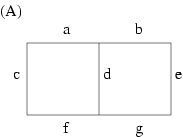There was a longer discussion over our second problem, using domino shuffling to prove that H(2,2,2,) = 20. The claim in the paper is, in order to count perfect matchings of these tiled hexagons, first "flatten" them into square grids, and then count. Give each matchings weights for example, for this graph (Figure A) we have cde + aef + bcg. Give weights of zeroes and ones, with zeroes to the vertical edges so that they don't contribute to the sum.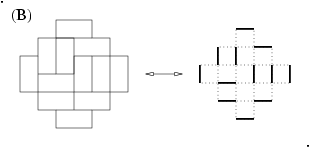Boytcho had a question about how do we fit these flattened hexagons into an Aztec diamond graph? NOTE: there IS a difference between an Aztec diamond and an Aztec diamond graph! (my name is gecko, not to be confused with geico, which can save....) See Figure B as an example to differentiate between the two.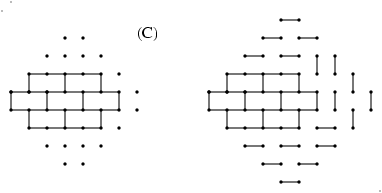Boytcho's question further asked, what weights would you assign to the flattened hexagons when trying to fit them into the Aztec diamond graph? Jim showed examples where it does and where it does not work to fit them in. Basically you must match the inner graph with the outer graph. See Figures C1 (not able to fit it into an Aztec diamond graph of order 4) and C2 (are able to fit it into an Aztec diamond graph of order 5). In C2, where the fitting DOES work, note the matchings that were NOT forced; there are only four possibilities between these, hence the "conclusion: the sum of the weights of the matchings of the graph is equal to four times H(2,2,2,)".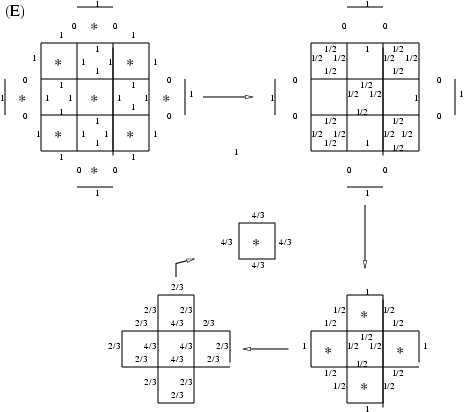Jim explained and gave two examples of the reduction algorithm. This algorithm is shown in the domino-shuffling handout, but Figures D1 and D2 show the step-by-step process of the examples that Jim explained. The second example is with a simple crushed hexagon to aid us with our homework. The basic procedure is to star every other cell, switch opposite weights on each cell, divide by the cell factors, erase the outer edges and then start the procedure over again. Finally you multiply all the cell factors and voila!; you wind up with the number of perfect matchings of the original graph.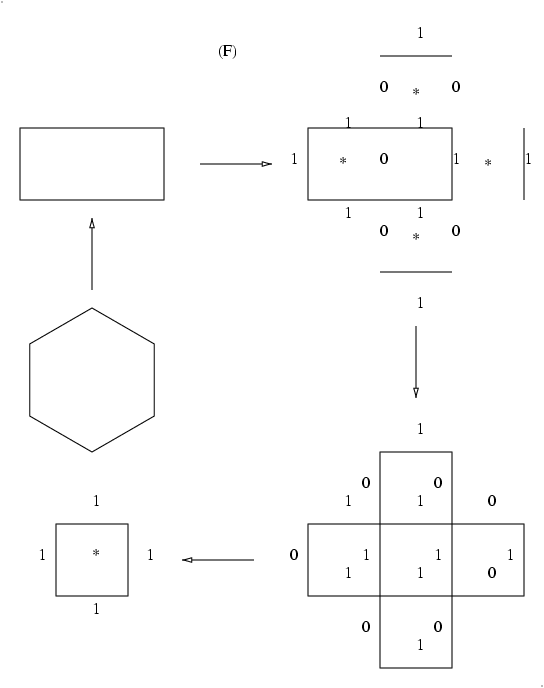We ended our session with Jim asking about a previous homework assignment, basically that we find questions and ideas about the Project Summary handout. We went around in a circle and these are the questions we listed (the questions that Jim answered have answers succeeding in square brackets, other questions to be answered in the future!):

## What should we do?

"What should we do?" [Read Sections 1, 2, 3, 4 of the generalized shuffling handout; read the entirety of the grant proposal; start programming if you can, programming neophytes should work on manual calculations; think about what other types of programs we want or need?]

Ergodic theory?

Positive and zero entropy regimes?

Will non-programmers be able to contribute? To whom can they talk? ['Computer mentors' such as Abe, Hal, Boytcho; also we can use our funding to purchase reference books for example, for programming.]

Pfaffian method?

When do we start? [Now! See Figure E and think about patterns, in this theoretical example and in examples of your own. In programming/coding, look for patterns!]

Aztec circle?

Role of wild ideas?

What should Hal program? (What should we program?)

Finally for all you vegetarians out there, Jim noted that cochineal extract is extracted from bugs, so if you plan to be a pure vegetarian, it is recommended to look for mosquito extract on fruit juice ingredients listings before you partake! (Thanks so much! Someday I will have so many things on my list of what NOT to eat that I will just constantly be spooning up tofu! How exciting!)

# SSL Minutes

15 Feb 2001
Boytcho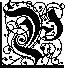avle will be the Beverage Person for Tuesday next.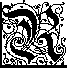achel said something about brownies.

## Intro to Ergodic theory

This is a theory which studies measurable dynamical systems. Being measurable means that said systems are probabilistic.

## Simple example: Fair Coin

If we throw a coin repeatedly we can talk about the probability of a sequence of events. For instance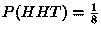Some Markov Chains also exhibit ergodicity. A simple MC is for instance: If it rains today then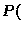it will rain tomorrow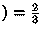, and if it is sunny today thenit will be sunny tomorrow.

The above examples have one dimensional time, but that need not necessarily be the case with ergodic systems. We can have two (, three, &c.) dimensions of time. For example see the domino tiling in fig 1.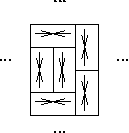Here we use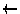to denote one of four possible states. The system depicted above has some built in restrictions. A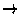state can only be to the left of asquare. A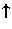state can only be under a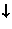square.

An Interesting Question is: How random can a system be with restrictions such as the above?''

Entropy is a measure of randomness.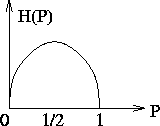The figure above illustrates entropy (denoted traditionally by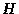for some reason), in the case of a coin. If the coin is biased so that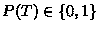, then there be no randomness, thus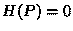. If the coin is fair, i.e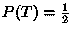then we have maximum entropy.

If we are taking measurements of a sequence of events (say repeated tossings of a coin) at the rate of one measurement per unit of time, the most randomness we can hope for is 1 bit per unit of time.

Then there was a brief discussion of Independent Identically Distributed events. The author of these notes is not quite certain, how to tie that in with the rest of the discussion, yet it seemed relevant enough to be mentioned.

## Another example

of a one dimensional system is the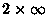square grid tiled by dominoes. A piece of it is shown in the figure bellow.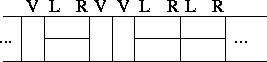In this case a restriction is that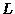and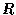states must be next to each other in the correct order. (There are exactly two tilings that we are neglecting here for their negligably low probability of occurring. Those are the two brick-work'' tilings). Here we prefer to use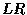for horizontal dominoes as opposed to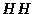, for this makes it a local rule, and makes it easier to verify if a piece of the tiling is correct. It takes a while to check if a long sequence ofs is of even length.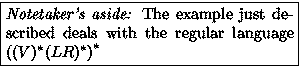So a question we want to answer is how random can a piece of that strip be?

If we start somewhere on the strip we want to know how likely are we to see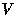in the next column as opposed to?

One way to make a random system of this kind is to use a biased coin to decide whether to put down aor an. If the bias-ratio is too close to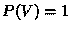(always) or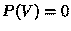(always LR), the system will have low entropy. It has higher entropy when the bias is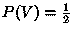(a fair coin), but this is still not the best choice. It turns out that the choice of bias that leads to the highest possible entropy is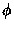(aka The Golden Ratio).

There is a close relationship between the Golden Ratio and Fibonacci numbers, namely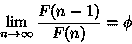in English: The ratio of two successive Fibonacci numbers approaches.

Now we can think back to the first homework for some insight on why this is a good bias for this particular coin. Consider a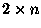piece of the strip. The first column of it can beor. If it bewe have a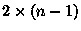piece to tile, otherwise the untiled part is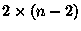. As we have seen the probabilities involved are related to the Fibonacci numbers.

Hal has seen matrices related to a similar system.

## In the 2-dimensional case

we can think of covering the plane with dominoes. One simple way to go about that is to divide the plane in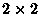pieces and use a fair coin to tile each of these pieces. We then have 1 bit of information to each, therefore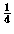bit per square. And we can do much better than that.

If we take a random tiling of a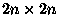square with dominoes, the probabilities of what domino covers a square in the inside are very much the same as they are in the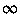-plane. We can ask for instance how likely are we to come across three horizontal dominoes, stacked one on top of another in a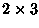. The situations would again be similar on a largeand the entire plane.

If we take however and Aztec Diamond, things go terribly wrong really fast. In the polar regions for instance the probabilities are skewed at places that are much further away from the border than in the simple square case.

In the very center of the Aztec Diamond, we have the max entropy.

We can use the Generalized-Domino-Shuffling (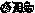) algorithm to find the probability of a stack of three h-dominoes by finding the number of matchings of a graph depicted below.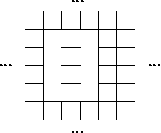If we chose to leave all the edges between the six vertices of interest as to simplify the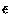calculations in, we would increase the number of matchings by a factor of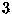.

In the Aztec Diamond, the polar regions are 0-entropy regions, whereas the temperate zone is of positive entropy.

If we travel from the center of the Aztec Diamond towards one of the poles, at about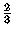of the way the probability of having a north'' domino approaches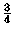.

Another phenomenon that may occur is Hole in The Wall''. This is a rectangle of even width, which if it is shaded in standard checkerboard fashion, has the white squares from the leftmost column and the shaded squares from the rightmost column removed. In other words, the sizes of consecutive rows alternate between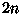and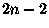and the rows are centered left to right. Such an animal has an unique tiling by dominoes.

The point of this is, that we may achieve the behavior of the sub-arctic region of an Aztec Diamond on a square if we remove the right pieces from the border.

Then we talked about halved Aztec Diamonds, and lattice paths in them, which force tilings.

Then Prof. Propp addressed a question by yours truly having to do with the basic moves on a Diabolo-tilable region. An appropriate illustration of the graph that we would want to match can be seen on p.10 of thepaper. The graph which consists of octagons and squares. The point is there are TWO basic move in the Diabolo world, one deals with the two matchings of a square, the other with the two matchings on an octagon.

The question was raised as to how to get a text file to fit predetermined line lengths in UNIX, which brings us to The UNIX Command of the Day: fold(1).

# SSL Minutes

20 Feb 2001
Hal and Nick

## Misc.

We were missing a couple of SSLers including Rachel, who was supposed to bring brownies. And we had a new guy, Joel. Hi, Joel.

There will be an "open bar" in the hallway on Thursday. We will drink up the excess beverages.

Clarification of previous notes:
Original: "maximum entropy is found at very center of Aztec Diamond"
Revised: there may be "bad spots" near center, but they'd be small enough to be negligible.
Why: Entropy is a statistical property, and we look at a small statistical region at the center of the diamond. If there is a bad spot, it is *statistically* small. Is that clear enough while still being accurate?

On Thursday, everyone will meet individually for 10-minute with Prof. Propp and Mike. A schedule has been devised. In the other room, we get to play on the computers and Abe will talk about *nix OSes and programming, the Hal will talk about his program.

Is everyone caught up on reading? Hal is not. Jim said that that can be a good thing, as long as you ware working on something else and asking good questions. For example, the "tilability question," or Boytcho's "Is there an analog to domino shuffling for diabolo tiling of a fortress?"

What are sticky points on the reading? "Why Generalized Domino Shuffling called GDS?" Also, someone asked about the notation in one of the papers.

## ASMs and Domino Tilings

A domino tiling of an AD can be described with a height function and put into a matrix.

      N          nN     nN = a north pointing domino
E N        wEnN
W E E  ==  wWeEwE   Ss = a south pointing domino
W N W       WnNeWe
S S         SsSs    E  = a east pointing domino
S           Ss     e

w  = a west pointing domino
W


In this picture, capitol letters represent black squares on a chess board and lowercase letters represent white squares. Obviously it is only necessary to enumerate which domino is the black tiles.

This is how Hal's been thinking about it, because that's how his program runs.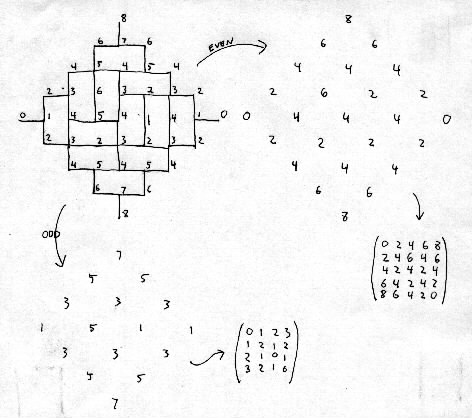This example takes even diagonal groupings:

A=(0 2 4 6 8
2 4 6 4 6
4 2 4 2 4
6 4 2 4 2
8 6 4 2 0)
multiply it by one-half (A*.5):
A=(0 1 2 3 4
1 2 3 2 3
2 1 2 1 2
3 2 1 2 1
4 3 2 1 0)
convert to ASM ('-' means "-1") of size (n+1)x(n+1):
A=(0 0 1 0
1 0 0 0
0 1 - 1
0 0 1 0)
Here's the ASM of the other (odd) diagonal grouping:
B=(0 1 0
1 0 0
0 0 1)
...which is a nxn permutation matrix.

C=(a b
c d)

ASM entry = { 0 if a+d=b+c , 1 if a+d=b+c-2 , -1 if a+d=b+c+2 }

Definition: ASMs are compatible if, when the small and large ASMs are converted to AD's, adjacent vertices differ by 1 or 3.

Question: For a given nxn ASM, how many compatible (n+1)x(n+1) ASMs are there?

Question: For a given (n+1)x(n+1) ASM, how many compatible nxn ASMs are there?

Number of positions free to change in smaller array is sum of "-1"'s in larger arrays ASMs.

Claim: An (n+1)x(n+1) ASM with k -1's is compatible with exactly 2^k nxn ASMs.

Claim: An nxn ASM with j +1's is compatible with exactly 2^j (n+1)x(n+1) ASMs.

Plot the locations of -1's in an ASM, and it might look like a circle, we think.

Bonus Questions:

(1) Show number of tilings of an order n AD that do not contain bad local conditions'' is equal to number of order n Baxter permutations.

(2) Prove the conjecture: the # of ASMs of order n that link up terminals as 1 to 2, 3 to 4, ..., (2n-1) to (2n) is exactly equal to the number of ASMs of order n-1.

Note: a -1 in the (n+1)x(n+1) represents something. ???

## The Square Ice Model

0 + 0 0       0 1 2 3 4
+ - 0 +   ==> 1 2 1 1 3 ==>  to the flux ==> rotated to get
0 0 + 0       2 1 2 2 2      line vector     densly packed
0 0 0 0       3 2 3 3 1      field.          flux line field.
4 3 2 2 0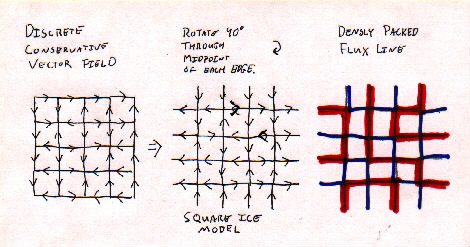I need a good explanation of what is going on here! Can anyone help?

Matrix to Vector Field

each entry of the matrix coresponds to a node, and neighboring nodes have an edge in the direction of the one of which the coresponding entry in the matrix is bigger.

Vector Field to Square Ice

Take each edge and rotate it by -90degrees ('-' here means clockwise.) The center of each square from the vf is now a node in the si picture.

Square Ice to Densely Packed Flux

Take the nodes of the si and color them chessboard-wise. Now look at the shaded nodes. The edges that go AWAY from a shaded node we shall color blue and the arrows that go INTO a shaded node we shall deem red. And then omit the directions for the dpf picture.

When looking at the dense flux line graph of an ASM, with, say, 12 terminals, (numbered 1 - c) the number of ways to connect terminals 2 & 3 is equal to the number of ways to connect 3 & 4. This theorem was proved by Ben Wieland.

        1  2  3
c
4
b
5
a
6
9  8  7


## Publishing:

There are three potential articles we have lined up:
1. Properties of tilings of different regions
2. Baxter permutations and domino tilings
3. Blue/green tilings

# SSL Minutes

27 Feb 2001
Dominic and Rachel

**Note: I apologize in advance if I did not understand/record your main points you presented on Tuesday. If I missed anthing, misinterpreted what you said, or said something you did not say, please send me a note explaining the mistake or what you would like changed.

Hal will bring the beverage for Thursday's meeting.

Kekule structures from benzene molecules are related to Hal's interest in nanotubes and their possible tilings. A benzene molecule yields 2 Kekule structures (2 perfect matchings).

Question: Do we want our meeting minutes in postscript or pdf?

We will collect the minutes of all our meetings into a separate file. It is a good idea to send out both rough and revised versions of the minutes. When revising minutes, though, send the addenda/errata and the full revision in separate messages. That way, people can immediately see what has changed from the rough draft, but also have a revised text to refer back to at a later date. Also, multiple revisions are allowed and even encouraged. Everyone can suggest changes, and the authors implement them.

Additionally people questioned how "good" should the minutes be? and should there be a difference in the quality of notes sent by Tuesday notetakers vs Thursday notetakers? The revision version of the notes should be as 'perfect' as we can get the notes, and that's how 'good' the notes should be!

We went around the room, and each person conducted the meeting for roughly five minutes.

Geir: Nothing in particular. Not so much interested in the Arctic Circle, but in the number of matching permutation matrices. Interested in Baxter Permutations. What are they?

**Homework assignment for Thursday: Find a definition of Baxter Permutations.

Abe: Concerned about the number of ways to join 1 & 2, 3 & 4, etc. Also interested in symmetries in blue/green paths used to join these numbers. Boytcho was also interested and had been working on these blue/green path symmetries as well.

Pavle: Interested in the tilings of a square (or diamond, depending on which way you look at it). Presented a few pictures to explain his ideas, and showed how he has been spending his time.

Dominic: Wondered why David Robbins tried to make his data look similar to Pascal's Triangle. It turns out Pascal's triangle can also be applied to Aztec Diamonds. Other than that, Dom has been spending most of his time reading the articles and learning computer language.

Nick: Opted to speak at the end of the meeting because his talk would probably take longer than 5 minutes. However, we did not have enough time at the end of the meeting for him to talk.

Joel: Has mostly been catching up. Interested in overlapping domino matchings, blue/green paths, and how they are related to superpositions of domino tilings.

Michael: Ceded his time to others.

Dan: Wonders if there's a good source to learn Java. Computer-literate people suggested taking Computer Science 368 here at the university. Also, perhaps read through a few Java books, and within a few minutes of reading, you should be able to tell if it fits your learning style.

Winston: Interested if there's research done in the tilings of any regular polygon. Jim showed the example of a regular octagon (edge length 1) being tiled by 2 squares and 4 non-square rhombi. There is no known way to tile a 2n-gon (I think). Also, Jim introduced ribbon tiles. There are two 2-ribbons. One looks like a horizontal domino. The other looks like a vertical domino. There are four 3-ribbons. They look like the Tetris pieces generated when one can only move eastward and northward. How many ways are there to tile a figure using ribbon tiles?

Rachel: Interested in having brainstorming sessions. Also concerned about what/how an SSLer without mathematical sophistication can contribute. Interested in having a study session, which could be facilitated if all members could post a list of their free times. The group decided it could be beneficial if a member specialized in one specific area, so even if he/she did not have mathematical or computer sophistication, he/she could teach others about his/her area of expertise.

Boytcho: I apologize, but I'm not exactly sure how to put this into words. He looked at all the different tilings of an order-2 Aztec Diamond. Then he translated each tiling into a 4-by-matrix. Then he transformed this into an arrangement of green and blue lines, assigned a "weight" to each colored segment, and looked for patterns.

Kristin: Has spent her time learning about computers. Interested in tiling different polygons. Can we use other shapes (which ones?) for tilings? When do you know if a region is tilable? Jim explained the marriage lemma and its connection with tilable/untilable regions.

Hal: Comments on the ideas Boytcho presented. He represents Aztec Diamonds as huge arrays. Interested in how we can go from a Aztec Diamond to any other shape. Hal's time also ran out and he will be given time at the next meeting to finish his minutes.

Figures will be added in the revision to these notes.

# SSL Minutes

1 March 2001
Hal and Boytcho

## People have things to say

Boytcho says that Mathematical Induction works for any well ordered set, such as the positive integers or the Cartesian product of a finite number of well ordered sets under the dictionary ordering. Someone forgets that the real numbers are not well ordered.

Hal asks what functionality should go into the program. He gets good feedback:

1. Detect large chunks of same type of block (aka icebergs)
2. Calculate Height Functions
4. Interactive and non-interactive modes.
5. convert to ASM (in real time)
6. Give lots of power to the interface.

Nick talks about how he's given some thought to representing a DPF (Dense Packed Flux). Example :

        o---o---X---o
|   |   X   |
XXXXXXXXX---o     4 6 8
|   |   |   | ==> 1 5 4
o---XXXXXXXXX     2 9 1
|   X   |   |
o---X---o---o

Each square is represented by a bitwise numbering system:
              1
o---o
8 |   | 2
o---o
4

Crash-course-type Intro to the Binary Number System: Traditionally humans use the symbols 0, 1, 2,... 9 to write numbers down. These are the ten digits. On those symbols is based the decimal (aka base ten'') number system. When we write down a number say 935, that means that the magnitude of it is obtained by the following formula 9*102 + 3*101 + 5*100 = 900 + 30 + 5. The position of each digit represents the power of ten that it is a coefficient for. We need exactly ten symbols for the base-ten representation of numbers to avoid a mess and have a unique representation for each number. Ten is a convenient number base for humans to work with, but not so for computers. They deal better with numbers represented in binary (base two). The symbols used are 0 and 1. (Traditionally to avoid ambiguity a subscript is used to indicate the base.) A binary number is 10012 for example. This is the number 1*23 + 0*22 + 0*21 + 1*20 = 910. The word digit is related to the decimal system in that it means one of the ten symbols used in it. Therefore with binary numbers we talk of bits (from Binary digIT). The numbers chosen by Nick above are all powers of two, which means that they have exactly one bit in their binary representation set to 1. Computers can easily be convinced to deal with numbers bit by bit. Another advantage of that choice is that the sum of the numbers corresponding to the on'' sides of a square never exceeds four bits, and its binary representation makes it immediately obvious which sides are on.''
Add together the numbers of those sides that have flux lines on them. Then you get a nice matrix. You can flip a square if it is a 5 or a 10. Let's flip the 5 in the center of the matrix to a 10. This affects the four squares adjacent to it. Legal moves:
        5 to a 10:       10 to a 5:
-4	              +4
+2 +5 +8	   -2 -5 -8
-1    	      +1

Then we get:
                               o---o---X---o
|   |   X   |
4  2  8       XXXXX---X---o
flip--> 3 10 12  ==>  |   X   X   |
2  8  1       o---X---XXXXX
|   X   |   |
o---X---o---o


Software

We get volunteers to look at redblue.c: Nick, Hal, Boytcho, Rachel. (http://jamespropp.org/hidden/ssl/blue-red.c)

We also need to find out what other software is out there: Abe, Nick.

## Baxter Permutations I (Boytcho)

Let N be an odd element of the Natural Numbers. Let IN = {1, 2, 3, 4, ... N} Let ON be the odd elements of IN. Let EN be the even elements of IN.

Definition: P is a Baxter Permutation iff

1. P(ON) = ON and P(EN) = EN
2. if P(n) is between P(2i) and P(2i+1) then n >= 2i
3. if P(n) is between P(2i) and P(2i-1) then n<= 2i

## Baxter Permutations II (Mike)

The set of permutations on In is called Sn. If w is an element of Sn, w is Baxter iff for every i in {1, 2, 3, ... n-1}, it passes the test.

The Test. Let r = w-1(i). Let s = w-1(i+1). Assume r > k. If there exists a ki such that, for all t in [k,r], w(t) <= i and, for all u in [s,k], w(u) > i, then the test is passed.

Here's a case by case run down:

1. If s < r Then we ask is there a k in [s, r] for which we know that P(s), ... P(k) are all <= i, and P(k+1),\dots P(r) are all > i?
2. If r < s Then we ask is there a k in [r,s] for which we know that P(r), ... P(k) are all > i, and P(k+1), ... P(s) are all <= i?
3. It is never the case that s = r.

Here is an example: we permute (1,2,3,4)--> (2,4,1,3). For i=1 we can partition in the following way 2,4,| 1. So we don't know yet this not to be a Baxter permutation. For i=2 we cannot however partition 2,4,1,3 in a way that would satisfy our question, thus we now know the permutation to not be a Baxter Permutation.

## ASMs and Monotone Triangles

There is a nice correspondence between ASMs and Monotone Triangles.

        0 1 0 0 0        0 1 0 0 0            2
1 - 1 0 0        1 0 1 0 0           1 3
0 0 0 1 0  <-->  1 0 1 1 0  <-->    1 3 4
0 1 0 0 0        1 1 1 1 0         1 2 3 4
0 0 0 0 1        1 1 1 1 1        1 2 3 4 5
ASM          Intermediate    Monotone Triangle

In each entry of the intermediuate matrix, add up the entries of the ASM going down the column until you get to the corresponding location. In other words, the (i,j) (row, column) entry in the intermediuate matrix is simply the sum from k = 1 to k = i of the (k,j) entries in the ASM.

Note that the rows of the intermediate matrix add up to (1,2,3,4,5)T.

Now, we ask the question: For each row of the intermediate matrix, which columns hold 1's?'' The answer to that is the monotone triangle.

Properties of the monotone triangle (MT).

              B
A <= B / \  B <= C
/   \
A-----C
A < C      

Usefulness: It is easy to generate MTs. (bluered.c)

## Plane Partitions and Semi-Strict Gelfand Patterns

What is a partition of a number? A set of positive numbers that add up to it. 10 = 6+4 or 10 = 5+3+2.

        Partition  Ferrer's Diagram
5          .....
3     -->  ...
2          ..


A Plane Partition has a three dimentional graph, not a 2 dimentional graph. Here's an example Partition:

        3 2 1                                1 2 3 7 8 9
2 1    --> Boxes in three Space. -->  1 2 4 7 8
1 2 5 7
1 3 6
1 4
1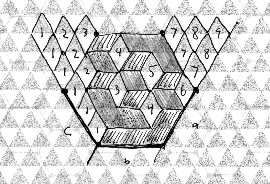Stack them as boxes inside a cube. Then think of that cube as equivilent to a hexagon tiles with lozenges. Draw that. Then extend the cornes out, as shown in the graph. Number the vertically oriented lozenges based on the distance from the line on the left side. Transfer the numbers into a triangle. That's a Semi-Strict Gelfand Pattern.

Properties of the Semi-Strict Gelfand Pattern:

            A < C
A-----C
\   /
A <= B \ /  B < C
B         

Why was that important?

# SSL Minutes

6 March 2001
Abe and Joel

For the record, Joel had his version of the notes to me promptly... I'm the slow one.

## I.

Jim gave a brief discussion on cyclic permutations and groups:

Transformations in the group MUST be rotations in the plane, not reflections or anything else.

There was a discussion of this applied to rhombus tilings, which I won't even TRY to draw in ASCII. Rotations in this case can only be rotated 120 degrees, giving an orbit of order 3.

Say there are m tilings that are invariant under cyclic permutations and 3n tilings that aren't. The total number of permutations would be m+3n. Removing the redundant ones would give m+n, which is unlikely to have a nice answer.

A usual question: "What is the number of ... up to symmetry?"

Changing symmetry can often simplify equations. Additionally, transformations can be paired, etc.

## II.

Another topic: Dense packing models of hexagon grids. Someone might want to look into this, as no one has investigated it yet.

## III.

Notes on programming: IT may be more efficient to make straightforward programs without worrying about nice ways of packing bits and so forth. This will make modification and extension easier.

## IV.

Coupling from the Past: It is difficult to generate a random ASM from scratch, but by repeatedly permuting in a random fashion, it will eventually reach something close to a uniform distribution. Coupling from the past is a method which is proven to accelerate this convergence.

## V.

Question: What about convex hulls around a set of points? How close is it to a circle?

## VI.

ASMs/TOADs: How does one create a dense packing from a TOAD? 1. superimpose two ASMs for TOAD 2. Turn these into Blue-green maps

We need some representation for TOADs and ASMs that will allow easy cycling through all variations. Semi-strict Gelfand Patterns are good for this application as they are easy to generate.

Date: Thu, 29 Mar 2001 22:59:54 -0600 (CST)
From: propp@math.wisc.edu
To: ssl@math.wisc.edu
Subject: correction to Notes for March 6

The notes for March 6 say:
>We need some representation for TOADs and ASMs that will allow easy
>cycling through all variations.  Semi-strict Gelfand Patterns are good
>for this application as they are easy to generate.


"Semi-strict Gelfand Patterns" should be replaced by "Monotone Triangles". Remember, Semi-strict Gelfand Patterns correspond to plane partitions; TOADs and ASMs correspond to monotone triangles. (Leastaways, ASMs correspond to monotone triangles; TOADS correspond to something a bit more complicated.)

Jim

technique: generation => convert to matching => examine

N is a function

\sum_A (1^{N(A)}) = number of ASMs
\sum_A (2^{N(A)}) = number of TOADs
\sum_A (3^{N(A)}) = number of nice function for some product formula?
\sum_A (4^{N(A)}) = UGLY (until nontrivial formula is proven)


Nice weighted product formulas are also important at times.

## Next Time:

Q analogs and P-adic (P is prime)

that's all.

# SSL Minutes

8 March 2001
Kristin and Nick

Jim requested that someone pick up an article that is in the Physics Library: Baxter, Kelland and Wu: J. Phys A_9, 397 (1976)

Jim also requested that we work on determining how to mutate a grid diagram by local moves in order to get all other possibilities.

## Geir explained how to use q-analogs.

He explained this in relation to lattice paths. Start with a grid and by moves of only down or to the left, go from upper left vertex to lower right vertex. How many paths are there? A way to represent the answer is to consider the area underneath the lattice path and encode the info with a polynomial in q. The coefficient of q^i equals the number of lattice paths with an area of i. So, in the example of a 2x2 square grid, the number of lattice paths can be given by: 1+q+2q^2+q^3+q^4.

Next Geir defined [n]=(1-q^n)/(1-q)=1+q+q^2+...+q^(n-1) He went on to define [n]!=[n][n-1][n-2]... and you can substitute for [n] in terms of the q notation previously defined. [n]! is called a q-factorial. This allows us to define q-binomial coefficients, which are analogous to the standard coefficents, but with the bracket notation. So, the q-binomial coefficient looks like this: [n]!/([n-k]![k]!)

This can be applied to the example of the 2x2 grid, where the number of lattice paths is given by !/(!!) And Geir worked this out and verified it.

So, in general, on the kx(n-k) grid, you use the q binomial coefficient to determine the number of lattice paths. Why is this useful? The q-analog gives more useful information. For example, it allows you determine how many paths there are for a given area under a path.

This can also be applied to the lozenge tilings of a semiregular hexagon with sides (a,b,c). We have a formula that tells us the number of tililings of such a region, which is the triple product of the quantity (i+j+k-1)/(i+j+k-2).

If we think of the three dimensional picture of cubes in a box that the hexagon tiling represents and take the triple product of [i+j+k-1]/[i+j+k-2] we get the number of cubes.

Jim went on to explain how q-analog can also be applied to the number of tilings of an aztec diamond. I am a little fuzzy on this though and Nick did not have much in his notes about this either.

Next we went next door and Hal showed us his really beautiful applet and Jim showed us another program which I do not know the name of and could barely see. There was also a 'tripped-out' program that was run for us.

# SSL Minutes

20 March 2001
Dan

(someone?) is working on proving that the larger matrix in the ASM representation of an order "2" (n) aztec diamond is the smaller matrix in the order "3" (n+1) ASM after doing domino shuffling.

Jim pointed out that "pushing down" the height in an aztec diamond is equivalant to adding [1, -1; -1, 1] to the ASM.

(Hal) proposed the idea of looking at ASM hieght functions with the minimum height fuction subtracted out of them to see if the properties were interesting.

Some ideas for the fair were tossed around, including displaying Hal's java program, and explaining various things we're working on, such as the Baxter conjecture and the blue-green model conjecture.

Jim explained one of the properties of compatable ASM pairs. Each ASM is compatable with 2**[number of -1s] smaller ASMs and 2**[number of 1s] larger ASMs. Each item can be represented as one item in an ordered postset, where each order of ASM represents one level of depth. By taking a random path from the bottom up you can generate a random pair of compatable ASMs. However, you need to use some sort of weighting to generate a random ASM because picking a random path will favor ASMs with more -1s

Geir showed that you can quickly convert between an ASM to a densely packed flux line model by going straight on 0 and turning on -1/1

# SSL Minutes

22 March 2001
Pavle

## What we did

We spent most of our time in the computer room, each doing a small project.

Hal was working on the heaxagon version of blue-red. That is, it will do for hexagonal tilings what it does for rectangular ones.

Joel was working on the probability of finding a 1 or -1 at a certain spot in a NxN ASM as a way to see if there is any way to get a circle, the likes of which we see on an Aztec diamond.

Pavle was working on figuring out a good algorithm to figure out which (N+1)x(N+1) ASM was compatible with which NxN ASM.

Geir and Kristin were computing the number of ASM's of N=1, 2, . . ., 8 to see how many of them connected 1 with the point futhest away on a tiling.

Dominic and Nick were doing research on baxter permutations, and trying to make a program that would let them know, for an input sequence, whether it was a baxter permutation or not.

Boytcho and Abe were working on figuring out a way to store a large quantity of numbers. Boytcho then pointed out that you can't store 20! numbers in less than a supercomputer, so that idea was shot down.

Dan looked at ASMs and the DPFL model. Also looked at the hex flux line model thingy.

# SSL Minutes

27 March 2001
Dominic and Hal

## Etcetera

Jim has assumes responsibility for spending the interest off of a $5000 endowment that a former undergrad has given the department to be spent on games. Any ideas? By the way, 5% of$5000 is \$250.

Joel will bring the beverage for Thursday.

The Undergraduate Research Symposium (URS) is quickly approaching. We need to decide what we will all have at the URS. We generated a rough list of topics, people responsible for different topics, and materials we need:

Topics

• what is it? (Hal)
• shuffling (Hal)
• lozenges (Rachel)
• ALTERNATING SIGN MATRICES
• definition (Geir)
• as DPFLs (Geir)
• CONJECTURES
• Baxter Permutations (Dominic and Nick)
• ASM shuffling conjecture (Boytcho)
• A(n-1) conjecture (also known as the proper linking conjecture)
• INTERESTS
• lozenge tiling flux lines (Rachel)
• probability of certain ASMs (Pavle)

Materials

• COMPUTER
• Jim will bring his laptop
• Hal will send a tarball with programs
• T-SHIRTS

When preparing a paragraph, picture, or presentation of the assigned topics, people should scan in necessary images, send the images to Boytcho, and send images and text to Rachel who will compile everything. Boytcho will make all of the images look nice with xfig.

Volunteers to attend the URS, and the times they are available:

• Hal: 9:00-10:00, 12:30-end.
• Kristin: 12:00-2:00
• Joel: 9:00-1:00
• Dan: 2:30-5:00
• Nick: 2:30-5:00

To show the relationship between Aztec Diamonds and ASMs at the URS, we do not have to explain height function or the algorithm used to find the ASMs from these heights. Instead, we can use a simpler method:

Look at the vertices in an Aztec Diamond that correspond to the entries of the larger (size n+1) ASM. Count the number of edges that meet at each vertex. This number will be a 2, 3, or 4. Subtract 3 from the number (this will yield a -1, 0, or 1). This produces an ASM from any tiling of an Aztec Diamond.

We can use a similar method to find the smaller (size n) ASM. Count the number of edges that meet at each vertex. This number will be a 2, 3, or 4. Subtract this number from 3 (this will yield a -1, 0, or 1). This produces an ASM from any tiling of an Aztec Diamond.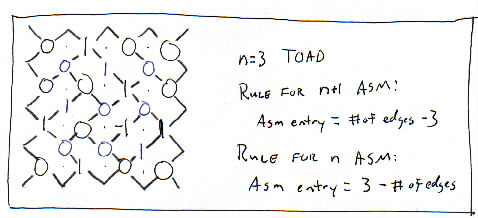## Mathematics

A Question (perhaps for Pavle): can we prove or even verify this conjecture?

Conjecture: If x and y are two different n-by-n ASMs, then the number of size n+1 ASMs that are compatible with both x and y is equal to the number of size n-1 ASMs that are compatible with both x and y.

In other words, if x and y are two different nodes on the same level of the ASM tree diagram, is the number of way to connect them by going up one level and back down is equal to the number of way to connect them by going down one level and back up.

Question: (posed by Joel and Geir) How many ASMs are there with only one -1? with two -1s? More?

Partial Answer: If there are A(n) ASMs of size n, there are A(n) - n! ASMs with one or more -1's in them. There are (n choose 3)2*(n-3)! ASMs with one -1.

Question: How many ways are there to go up k levels, starting from the bottom? (1,2,8,80,...) Maybe there's a nice theorem lurking there.

Jim's article titled "The Many Faces of Alternating Sign Matrices" is due by April 20th. So everybody should prove important stuff by then.

That leads to a Conjecture: The number of domino tilings of an n-by-2n rectangle is always odd. (Can we prove this by showing that for any n, we can match Dominos into pairs with a remaining singleton?)

Abe asks about Components and Linkages. He wants to know a good way to count the number of components of a matching. Jim gives a good answer: First, see what even number 1 matches with. Next, move one place to the right. See what even number this number matches with. Again, move one place to the right. See what even number this number matches with. etc.

## Baxter Permutations

Dominic: What's the deal with the two different definitions of Baxter Permutations? For example, one definition works only on odd numbers. Another definition works on any number. How do these two definitions relate?

Note: Hal will introduce some notation into the notes. I hope this helps.

The object of interest here is an order n Aztec Diamond where both of the corresponding ASMs are permutation matrices. (An ASM without -1's is a permutation.)

If we take a size n+1 permutation matrix, there is exactly one size n compatible ASM, because we know that the number of of smaller compatible ASMs are 2^(# of -1s in the bigger ASM) = 2^0 = 1. This smaller ASM may or may not be a permutation matrix. If it is a permutation, then we conjecture that the size n+1 ASM is a type II Baxter permutation.

What is the difference between a Type I Baxter Permutation and a Type II Baxter Permutation? A type I permutation is odd in size (2n+1), and sends odds to odds and evens to evens. If we take out the odd elements of the type I Baxter Permutation (1BP) we get a size n+1 Type II Baxter Permutation (2BP). We think that each 2BP corresponds with exactly one size n permutation that, when put together, form a 1BP.

According to the Baxter Permutation Conjecture, each size n+1 2BP is identical to a size n+1 ASM without -1's whose single compatible size n ASM is also has no -1's. Furthermore, if we construct a permutation matrix Pi,j of size 2n+1 where if i and j are odd, they correspond to entries of the size n+1 ASM and if i and j are both even, they correspond to entries of the size n ASM, and if i and j are not both odd or even, the entry is zero; then P is a 1BP corresponding to the original 2BP.

Example 1

+ 0 0     0 + 0 0
0 + 0 and + 0 0 0 are compatible.
0 0 +     0 0 + 0
0 0 0 +
As permutations:
2 4 6  and 3 1 5 7 
We can rewrite that by interspersing the ASMs:
0   +   0   0       0 0 + 0 0 0 0
+   0   0         0 + 0 0 0 0 0
+   0   0   0       + 0 0 0 0 0 0
0   +   0    -->  0 0 0 + 0 0 0   -->   3 2 1 4 5 6 7
0   0   +   0       0 0 0 0 + 0 0
0   0   +         0 0 0 0 0 + 0
0   0   0   +       0 0 0 0 0 0 +    
For example: 3 1 5 7 (1 maps to 3, 3 maps to 1, 5 and 7 map to themselves) induces 2 4 6 (each maps to itself). Thus we have 3157 and 246. But we can write these two permutations as one larger permutation as follows: 3214567.

Question: How does a size n+1 2BP induce'' a size n permutation?

Example 2

0 0 +     0 0 0 +
0 + 0 and 0 0 + 0 are compatible.
+ 0 0     + 0 0 0
0 + 0 0
As permutations, we can write them as 3 2 1 and 3 4 2 1. We claim that 3 4 2 1 is a 2BP. We can construct a 1BP from these two permutations:
3 2 1, 3 4 2 1 --> 6 4 2, 5 7 3 1 --> 5 6 7 4 3 2 1

Conclusion: We seem to have missed the big clue that was set out in front of us: the existence of two types of Baxter Permutations, along with the fact that a TOAD corresponds to two ASMs.

# SSL Minutes

29 March 2001
Dominic Johann-Berkel

## Baxter Permutations:

Dom presented information about Baxter Permutations:

In 1954, Eldon Dyer asked the following question: Let f and g be continuous functions that commute under composition (that is, f(g(x)) = g(f(x)) for all x). Let f and g map the unit interval [0,1] into itself. Must f and g have a common fixed point, meaning a point z such that f(z) = z = g(z)?

Though William Boyce ultimately proved this false, the investigation of Dyer's conjecture yielded interesting information. Specifically, if f and g do have a fixed point (in fact, if they have finitely many fixed points), then the pair of commuting functions induces a Baxter permutation.

If N denotes an odd natural number, IN denotes the set of natural numbers through N, ON the odd elements of IN, EN the even elements of IN, and P is the permutation P: IN --> IN, then we can define a Baxter permutation as follows:

Def: P: IN --> IN is a Baxter permutation (Type I) of order N if and only if
1.) P(ON) = ON, P(EN) = EN;
2.) If P(n) is between P(2i) and P(2i + 1), then n = 2i;
3.) If P(n) is between P(2i - 1) and P(2i), then n = 2i.

(Note: This is the definition of what we have been calling a Type I Baxter permutation. At this point in my presentation, I should have defined a Type II Baxter permutation since the rest of my talk was primarily concerned with Type II Baxter permutations.)

Following the definition, I argued (admittedly, rather weakly) that there was a correspondence between Baxter permutations and thee non-intersecting paths. This correspondence consists of composing two bijections. The first one joins Baxter permutations and twin binary trees, the second one joins twin binary trees and three non-intersecting paths.

For the sake of simplicity, I will reconstruct my argument through a different (better) example. In class, I applied my argument to the following Type I Baxter permutation: 3 2 1 4 5 6 7. The paper which outlines the argument uses Type II Baxter permutations. Thus, I will proceed by examining a Type II Baxter permutation.

Def: A permutation P of Sn is a Baxter permutation (Type II) if and only if, for all integer m?[n - 1], P can be uniquely factorized by: P = P'.m.P<. P>.m+1.P'' or P = P'.m+1.P>.P<..m.P'' Where all the numbers of P< (respectively P>) are less than m (respectively greater than m + 1).

Thus, according to this definition, 4 2 3 6 5 7 1 is a Type II Baxter permutation of order 7. For example, for m = 4 we have P< = 2 3 and P> = 6. For m = 1, we have P> = 3 6 5 7 and P< = X. Etc.

Lemma: There is a bijection between Baxter permutations (Type II) on [n] = {1,2,,n} and twin binary trees. Proof: For simplicity's sake, I omit the proof. To see a copy of the proof, please see (S. Dulucq, O. Guibert, Mots de piles, tableaux standards et permutations de Baxter, 6th Formal Power Series and Albegraic Combinatorics Conf., Dimacs, 1994, Discrete Math., to appear).

Ex: Look at the Type II Baxter Permutation 4 2 3 6 5 7 1. According to the Lemma, there is a bijection between this permutation and a pair of binary trees. We show this bijection through an example:

           4 2 3 6 5 7 1

1            7
/ \          / \
2            6   1
/ \          / \  |\
4   3        4   5
/ \ / \        \  |\
5        3
/ \      / \
6   7    2
/ \ / \  / \


To form the first tree (the increasing tree), we start with the least value in the permutation, 1. Then we see where the next value, 2, is in relation to the one. It is to the left, so we draw a stem downward to the left. Next, we see where 3 is in relation to 2. It is to the right of 2 and still left of 1, so we draw a stem downward and to the right from 2. Next, where is 4? It is to the left of 3, but it is also to the left of 2. Thus, we will draw a stem downward and to the left from 2. 5 is to the right of 3. 6 is to the left of 5. And 7 is to the right of 5. Since each number in the binary tree must have two descending branches, one to the left and one to the right, we can add "leaves" to the binary trees as shown by the dotted lines. Thus, every number has either two descending stems, one descending stem and one descending leaf, or two descending leafs. To form the other, decreasing, binary tree, we start with the greatest value in the permutation, 7. We repeat the same process until we form another tree. The increasing and decreasing binary trees form a pair of twin binary trees.

Lemma: There is a bijection between binary trees and pairs of non-intersecting paths (there are more details, but for the sake of this discussion, this much will suffice).

Proof: Again, for simplicity's sake, I will omit the proof. See (S. Dulucq, O. Guibert, Baxter Permutations, 1998, Discrete Math., 180, 149).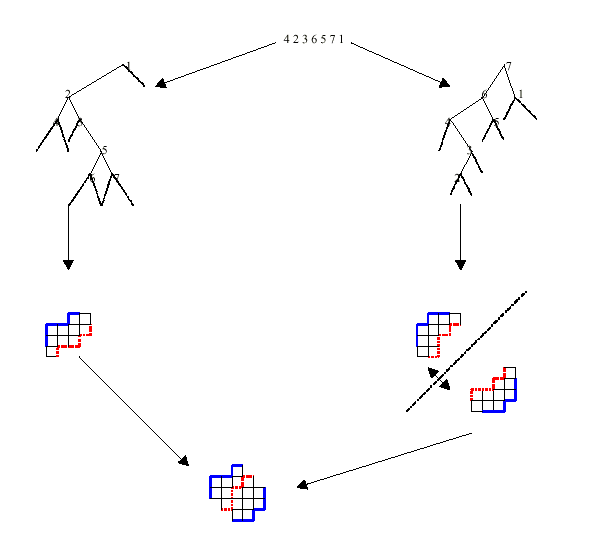This correspondence is equivalent to travel in prefix order the completed binary trees and to code, respectively, the internal edges and the leaves. The first paths (shown in blue) are obtained by coding the internal left (respectively right) edges by a North (respectively East) step. The second paths (shown in red) are obtained by coding the left (respectively right) leaves (except the two extreme ones) by an East (respectively North) step.

Thm.: There is a bijection between twin binary trees and three non-intersecting paths.

Proof: According to our previous lemma, twin binary trees correspond to a pair of two non-intersectingThat about does it. That is what I attempted to demonstrate during Thursday's meeting. In hindsight, I would have done quite a few things differently, but I hope the preceding explanation helps clear things up. For information related to Baxter permutations, or to see more rigorous demonstrations of what I tried to talk about, please see any of the following articles:

W.M. Boyce, Generation of a class of permutations associated with commuting functions, Math Algorithms 2 (1967), 19-26.

S. Dulucq, O. Guibert, Mots de piles, tableaux standards et permutations de Baxter, 6th Formal Power Series and Albegraic Combinatorics Conf., Dimacs, 1994, Discrete Math., to appear

S. Dulucq, O. Guibert, Baxter Permutations, 1998, Discrete Math., 180, 143-156.

F.R.K. Chung, R.L. Graham, V.E. Hoggatt, M. Kleiman, The number of Baxter permutations, J. Combin. Theory Ser. A 43 (1986) 1-22.

I have more articles if you're interested, but these are the most interesting ones.

# SSL Minutes

5 April 2001
Nick Pongratz

## Debriefing of Undergraduate Research Symposium:

• Overall spectator attendence seemed a bit low.
• During the debriefing, Nick learned a thing or two about the grammatical use of "good" vs. "well"
• Tips for next time:
• Make the posters more visual (more cool images, less "boring" text)
• Don't wait till last minute.
• We then went around the room for ~4 minutes each:

Abe: Was thinking about the A(n-1) conjecture. How to solve? - Find a bijection. - Boytcho: fill in edges of blue-red DPFL, flip coin to determine where to place horizontal lines, vertical lines are then forced.

Dominic: Will send out to the list the algorithm to generate Baxter permatations of any order n. (specifically, Hal, Boytcho, Nick wanted it.) Would like ideas on how we'll prove Baxter conjecture.

Nick: Working on a program that determines if a given permutation is a Baxter permutation. After that, will start coding the Baxter permutation generator.

Kristin: "Where is everyone?" Asked Abe if he has a program to count linkings of DPFLS's. Abe said the program is almost done.

Dan: Would like to get involved with a project with Hal and Rachel. (Which project is this?)

Boytcho: Has been looking to unify the two definitions of Baxter permutations. Jim says we should think about height functions when thinking about proving the shuffling conjecture.

Hal: Wanted to know what the final version of his applet should do: Boytcho suggested including a way to manually make elementary moves. Abe suggested it show the height functions. Debate over implementation ensued. Should we have toggle buttons or separate versions as we add features? Jim would like a "turbo mode with autopilot" and a tutorial mode. Michael suggested the code be modularized.

Jim encourages us to set up our own focussed meetings.

Everyone should meet with Michael next week (April 9-13) for 15-45 min. Email him a time that will work well.

• # SSL Minutes

10 April 2001
Boytcho Dontchev Peytchev

## Misc.

Boytcho may need to be reminded about the bringing of beverages for today.

We need T-shirts to be designed. Ideas that were expressed:

• pretty pictures with the code that produced them as a background
• the cochineal animal
• as there is a history of T-shirts involving images that relate in one way or another to what we're doing the picture of the hexagonal DPFL was brought up as a novelty.

In order to talk about things we need a nice way to name them. Among other reasons there's also the reason of having things published.

Worked on the Proper vs. Rigid linkings. Geir suggested that we call the proper linking "sequential". We can represent each of the pairings as lattice paths and it was suggested that we call the proper linking "Short" and the rigid linking "Tall".

The record shows, that prof. Propp will ask John Conway about names for things.

## Then there were round robin, What have i been up to?''

Kristin was working with Abe on code that counts specific pairings of DPFLs

Boytcho was playing with Baxter Permutations

Joel is working on what was referred to as "reduced DPFLs" or maybe "even DPFLs" which arise when the black vertices are removed from a chessboard colored graph with a DPFL. One question is how much info is lost in the transition from the complete to the reduced DPFL.

Dan has managed to avoid paying attention to his classes with the help of honeycombs.

Jim is trying to extrapolate the number of tilings of a 2xn rectangle (by dominoes, ribbons, whathaveyou) into the realm of negative n.

Geir is looking at the A(n-1) conjecture with both ASMs and DPFLs

Hal's been hacking the domino shuffling thing.

Nick is working on a BP verifier and wants to get to a generator based on that.

Dominic is working on a different (?) BP generator.

At this point it was suggested that we may want to look into things that BARELY FAIL to be BPs and TOADs with very few -1 in the corresponding pair of ASMs. Is there any correlation in the two?

The question was raised if ppl have difficulties accessing the literature. If so Prof. Propp and Michael Lang may be able to facilitate that on a case by case basis as ppl contact him.

It was observed that an Annotated Bibliography would be A Good Thing. Geir was appointed chief maintainer of said Bibliography.

Then we made a List. The List was of Areas Of Interest in the group. Neither five, nor seven are of them, yet there are six as follows:

1. the A(n-1) Conjecture: Geir, Abe
2. The Baxter Permutation Conjecture: Boytcho, Dominic, Nick, Hal, Geir
3. Hexagonal DPFLs: Dan, Boytcho, Rachel, Hal
4. "even" DPFLs: Joel
5. Software: Hal, Boytcho
6. Specific pairings: Kristin, Boytcho, Abe

The subsets of SSL outlined above, should schedule meeting times between themselves to work on the things they find of common interest.

In B107, Michael showed us a couple programs which are on dodgson: vaxrandom and printtiling. These programs can be found in Jim's directory. vaxrandom is a program which allows you to tile virtually any region you tell it to, While printtiling will make a pretty PostScript file out of the plaintext given by vaxrandom

another thing that was mentioned was drawtile.

some net-resources that are of use are:

People who find themselves lacking software that would help them get things done that they want done should contact Michael, who would then relate people's desires to the Coders among us.

have fun
B.

# SSL Minutes

12 April 2001
Geir Helleloid

Pavle has left us for the rest of the semester. Goodbye Pavle.

Question: Given a random ASM, what is the average value in a given matrix position (where the average is weighted by the number of paths to the ASM in the shuffling lattice)?

Example of how to run a process in the background:

Create a file - let's name it "runbr" - and make it executable. (chmod 755 runbr)

In runbr, have one line, e.g,

	blue-red 10 > br10

Then start the process with:

	nohup runbr &

Question: What happens if you convert TOADs to single ASM's (by interleaving the entries with zeroes and getting an ASM of order 2n+1)? Are there any patterns you can find (in the DPFLs, etc.)?

After this question, Hal began his presentation on his proof of the Baxter Permutation Conjecture.

After Hal spoke, we adjourned to the computer lab, where we did our own stuff.

# SSL Minutes

17 April 2001
Nick Pongratz

We had two guests, Dan and Chris.

We went around the room getting caught up on everyone's progress:

Michael- is polishing part of his dissertation.

Abe- has been listening to Hal explain his proof. is soliciting comments for brhelper.

Jim- 1: working on article, "Many Faces of ASMs," would like to put in a section, "Pairs of ASMs" if Hal's proof is convincing. 2: another article, "Z^2 Action". 3: has been playing around with hexagonal DPFL's 4: Terminology: DPFL should maybe be called Fully Packed Loops. Rachel would like to call DPFLs Aztec Phoenix Feathers. Everyone likes the terms "proximal" and "distal"

Dominic- explained how a counterexample to Hal's proof does not work. is going at the Baxter Conjecture differently than Hal.

Hal then explained to our guests what we're doing with Baxter Permutations, ASMs, and TOADs.

Nick- is taking Mike's advice with the Baxter Permutation verifier, will simply separate each element on the commandline by a space (IOW, let UNIX and C do the tokenizing). has worked on that a little bit since the weekend.

Geir- working on A(n-1) conjecture. typed up unannotated unlinked bibliography.

Kristin- last week played around with computers with Michael. looking for patterns of different growth rates. Was given the advice that most UNIX shells offer the command "factor" and Maple has "ifactor", both of which factor a number into primes.

Dan- had all of his exams on one day. Brutal.

Joel- hasn't done much over the holiday weekend.

Rachel- found there are no "rigid" pairings of hexagonal DPFLs.

Hal- Explained his proof for the Baxter conjecture. Didn't completely finish, will finish at next meeting.

# SSL Minutes

19 April 2001
Dominic Johann-Berkel

Nick will bring the beverage for next Tuesday's meeting.

We discussed what we would like to have on our shirts. Possible ideas:

1. Hexagonal FPL
2. ASMs with a superimposed Aztec Diamond
3. Show everthing (TOAD, ASMs, DPFL, etc.) so people can see how they are related.

It was decided that Dan and Joel (since they were not present at the meeting) will be responsible for organizing, choosing, and producing the shirts. Ha. Seriously, if anybody has an idea for a shirt design, make a mock up design to present at Tuesday's meeting. Otherwise Jim and Mike will draft somebody to head the shirt project, or we might scrap the idea all together.

Hal announced that he will be giving a talk on Monday at 4:30 in room 2321 Sterling Hall (the Physics Club room) regarding undergraduate research. Coffee and cookies will be served.

Hal continued with his proof of the Baxter Conjecture. He outlined his proof for the second half of the conjecture. That is, Hal showed that, when viewed as a permutation matrix, a Baxter permutation in the larger ASM and the existence of a -1 in the smaller ASM are the same thing.

Dominic then presented a different method of approaching the Baxter Conjecture. While Hal's method works from the outside inward, Dominic used a method that worked from the inside outward. That is, he used Hal's method to determine all the zeros of the smaller ASM. Then he looked at all the remaining "undetermined elements." There are three cases:

1. The undetermined element is flanked by two 0's and two 1's.
0   1
x
1   0

In this case, the undetermined element must be a 1. If it were anything other than a 1, there would be no possible way to tile the area with dominos; there must be a 1-by-1 square somewhere.

2. The undetermined element is flanked by three 0's and one 1.
0   1
x
0   0

In this case, the undetermined element must be a 1. If it were a -1, then there would be no possible way to tile the area with dominos; there must be a 1-by1 square somewhere. The undetermined element cannot be a 0. To see this, we must examine three cases. In each case, a shape is forced to propagate to the edge of the Aztec Diamond. But this shape does not fit the edge shape of an Aztec Diamond. Thus, we have a contradiction, and the undetermined element cannot be 0.

3. The undetermined element is flanked by four 0's.
0   0
x
0   0

In this case, the undetermined element cannot be a 0. To see this, we assume it is a 0 and derive a contradiction. We must examine four cases. In each case, we propagate a shape through the larger ASM until we hit a 1. Then the shape changes to a shape that continues propagating to the edge of the Aztec Diamond. But this shape does not match the edge of an Aztec Diamond. This is a contradiction, so the undetermined element cannot be a 0.

Thus, we can always determine all the 0's of a smaller ASM. We can then alternate between 1 and -1 for the undetermined elements in a given row or column, and proceed with the proof as Hal described.

Next, we went around the room to see what people had accomplished since Tuesday's meeting.

Abe: nothing
Dom: the above method of proving Baxter
Nick: nonBaxter stuff
Geir: not done much since Tuesday
Kristin: not done much since Tuesday
B'cho: not done much since Tuesday
Hal: Baxter stuff

We then retired to the computer lab where we each worked on our own stuff.

# SSL Minutes (Part 2)

19 April 2001
Nick Pongratz

Here's the few notes I took dealing with the programming of reusable components for further research:

We want an ASM class.

Variables: storage array (char)
size of ASM

Constructors:
fromMonotone
fromHF
fromPermutation
fromFPL

Methods: find_bigger_compatible( *function(ASM))
find_smaller_ "        "        "
display
elementary moves


That's all I have. I know we talked about more stuff, but my notes kind of drift off. What else do we need? What do we have implemented, Boytcho?

# SSL Minutes

24 April 2001
Rachel and Geir

Geir and Rachel took notes.

We had another visitor, a post-doc, called Rebecca. She is a possible candidate for leading SSL next year in Jim's absence.

We talked about tee-shirt ideas. No one had designed a concept over the weekend and with the end of the semester coming we are getting desperate! Rachel, Kristin and Dominic were willing to make the final decision of what to put on the shirt and get it all together to be sent out. Geir and Hal said they would put together their own concepts to give to us for Thursday's meeting. Mike suggested we use an idea from the Baxter conjecture and proof since that seems to be the only major new things completed this semester. Other ideas generated from the group are random tiling an actual toad! to combining matrices and tilings in layers on the shirt (although the tilings have been on previous shirts, so it wouldn't necessarily show new things accomplished this semester). One thing or another, we need to get cracking on this, and choose a concept for the shirt by the end of this week!

Things to work on in research: PARTIAL RESULTS should be written down and sent to the rest of the group. This is beneficial to everyone in the group! For example, it helps to prevent repetition and making the same mistakes as someone else in the group who already did. Please send out partial results!

Additionally, send out QUESTIONS that you want to be answered that you cannot answer yourself. Nick volunteered to keep the question list, so please send your questions to Nick.

Mike sez:
Please don't restrict yourself to questions you can't answer yourself. I think it would be nice if this list had all the questions you can think of to ask, whether you've tried to answer them or not. If you have, then include whatever progress you've made. I'll send out another example in the next message.

There are some modifications mentioned to Hal's applet. Dan volunteered to help. An example of these would be to outline the open squares that come in the shuffling of the applet so that the viewer will more easily see what's going on.

Mike sez:
Another mod would add arrows to the tiles to show which direction they will move.

There was a question related to the An-1 conjecture about connections from the first terminal to the 2n terminal and "reeling in" the linking. I had forgotten I was taking notes and forgot to write down what the actual question was. (Mike?) No one volunteered to work on this one.

NOTE: next WEDNESDAY MAY 2nd our group is speaking to VIGRE at noon! Think about what you can do to participate in this, if you want to discuss your research, etc.

Progress reports:

Dominic is studying on path connections on an ASM leading to an undetermined element.

Nick is programming, looking at non-Baxter stuff.

Dan ?

Geir is working on the An-1 conjecture.

"Baxter boy" (Hal) is putting his proof on paper.

Kristin is comparing data on FPLs.

Boytcho has been sick, has not been able to do much.

Rachel is putting together her hexagonal proofs, will write them up to send to the group.

# SSL Minutes

26 April 2001
Hal

Jim Asks what Pavle passed on to people. Should we check the notes?

Jim asked about the up-down conjecture. We told him that the next big programming push will be to check that out.

Kristin should email out her results (linking probability numbers) soon (DONE).

Question: What are the average number of components of the DPFL/FLP/EIEIO matchings? Abe will look at that.

That led to another question: What is the proper way of counting components? There are two natural answers:

1. Break the DPFL/FLP between 2n and 1.
2. Take the max number of components that can be produced by breaking the DPFL/FLP at any place.
How was it done in that theorem for lattice paths?

We heard a story about where the gyrational symmetry came from. The theorem was first stated as something that was obviously not true all the time, but then it turned out that it was. The moral of our story has something to do with our intuition.

I forced Rachel to take a stand on whether she believes in gyrational symmetry for Hexagons. She says no, but was unwilling to place money on it.

Rachel then talked about her theorem about the lack of what would be a distal matching of an ASM-DPFL in hex-DPFLs. The thing that Jim called a hole in her proof has been proved, but Rachel did not explain how she filled it very well. But I understood.

We talked about the stupid t-shirts.

The Last Supper will be on the 17th at 6pm. We will not be eating at Domino's Pizza. Somebody noted that we have the right number of people for the last supper (12+1).

## The VIGRE Lunch

Wednesday the 2nd at 12:00pm.

The whole week after this (6-12th), Jim will be in the Black Forrest.

Back to the lunch: Topics will include the Baxter theorem and HexDpfl's. Jim will talk, then he'll open things up for general discussion.

I ask about local moves. Jim says a good way to check would be with the 7 proximal DPFLs of a given size.

Abe can get to work on programming now that he's finished the paper he had to write.

Nick wants a web account. I got out my magic wand and gave him one.

Dominic wants to work on the big size 2n+1 ASM that comes from smushing two compatible ASMs together.

## The Razamov-Stroganov Game

Here is the game: First, pick an N. Then pick a size N square DPFL: we'll call this one the target. There are two players.

• Player A randomly chooses a DPFL. He wins if his DPFL has the same matching as the target.
• Player B randomly chooses a DPFL. She then makes exactly one random mutation to the matching from her DPFL. If the resulting matching is identical to the target's matching, she wins.

Definition of Mutation: pick two adjacent terminals i and (i+1)mod(2n). suppose i connects to j and (i+1)mod(2n) connects to k. Reconnect i to (i+1)mod(2n), and j to k. It is possible that i might equal k to begin with, in which case this mutation changes nothing.

Conjecture: Players A and B have the SAME chance of winning!

Proof for N=3.

There are 5 matchings for this size: two are proximal (P1 and P2), and three are distal (D1, D2, and D3)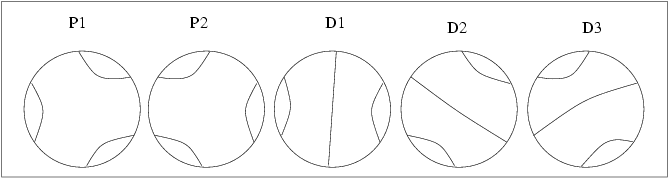Table of probabilities:

      P(A)      P1  P2  D1  D2  D2     P(B)
-----------------------
2/7  P1 | 3/6 0/6 1/6 1/6 1/6 |  2/7
2/7  P2 | 0/6 3/6 1/6 1/6 1/6 |  2/7
1/7  D1 | 2/6 2/6 2/6 0/6 0/6 |  1/7
1/7  D2 | 2/6 2/6 0/6 2/6 0/6 |  1/7
1/7  D3 | 2/6 2/6 0/6 0/6 2/6 |  1/7

Look at this as a matrix equation:
      2/7     3/6 0/6 1/6 1/6 1/6   2/7
2/7     0/6 3/6 1/6 1/6 1/6   2/7
1/7  =  2/6 2/6 2/6 0/6 0/6 * 1/7
1/7     2/6 2/6 0/6 2/6 0/6   1/7
1/7     2/6 2/6 0/6 0/6 2/6   1/7


This relates to the all-pervasive eigenvalue problem.

Homework: Do this for n=4.

From propp@math.wisc.edu Tue May 1 13:45:00 2001
Date: Tue, 1 May 2001 13:28:38 -0500 (CDT)
From: propp@math.wisc.edu
To: hwcanary@students.wisc.edu
Subject: homework assignment

I think you asked for this:

Read Razumov and Stroganov's new article! And then see if you believe my new

Conjecture: the relations given by their Conjecture 1, in combination with the fact that each distal linking-pattern arises from exactly 1 ASM, and in combination with the symmetry property proved by Wieland, suffice to determine the number of ASMs that give rise to each linking pattern, and in particular, the total number of ASMs.

Proof for n=3. There are only two geometries that a linking-pattern can have in this case: proximal and distal. Let P (resp. D) be the number of ASMs corresponding to any given proximal (resp. distal) linking-pattern. Pretend for the moment that we don't know P and D. Since there are 2 proximal and 3 distal linking-patterns, the total number of ASMs is N = 2P+3D. If the fixed link-pattern that the players are aiming for is the distal pattern 1<-->6, 2<-->5, 3<-->4, then player A has a chance of winning equal to

(*)	D/N


Now let's compute player B's chances. For each of the five linking patterns that he could have started with, I give the probability that he could end up with 1<-->6, 3<-->4, 5<-->2:

1<-->2, 3<-->4, 5<-->6 (proximal): 1/6
1<-->2, 3<-->6, 5<-->4 (distal):   0/6
1<-->4, 3<-->2, 5<-->6 (distal):   0/6
1<-->6, 3<-->2, 5<-->4 (proximal): 1/6
1<-->6, 3<-->4, 5<-->2 (distal):   2/6

So B's chance of winning is

(P/N)(1/6)+(D/N)(0/6)+(D/N)(0/6)+(P/N)(1/6)+(D/N)(2/6)


or

(**)	(P/3+D/3)/N


Comparing (**) with (*), we see that the RS conjecture implies

	P/3 + D/3 = D


which reduces to

	P = 2D.


But now we pull out the fact that each distal linking-pattern arises from exactly 1 ASM. This gives us D=1, and the formula P = 2D now gives us P = 2, so we've calculated everything we want to know, and the number of ASMs of order 3 is N = 2P+3D = (2)(2)+(3)(1) = 7.

Homework (everybody!): For Tuesday, determine whether my conjecture is true for n=4.

Keep in mind that Ben Wieland has a different conjecture, which relates A_n(pi) to A_{n+1}(pi') where pi is a linking-pattern of order n and pi' is a linking pattern of order n+1. Even if the Razumov-Stroganov relations don't suffice to force the values of all the A_n(pi)'s, maybe they do when taken together with the Wieland relations (or other relations that you guys are about to discover!).

And if we can find enough relations to force the values of all the A_n(pi)'s, then we're halfway towards discovering (among other lovely things) a new proof of the original ASM conjecture, totally different from both Doron Zeilberger's proof and Greg Kuperberg's proof!

Jim

We retired to B107, and I made a pretty hexDpfl picture for the stupid t-shirt. Don't know what others did. Then Rachel, Boytcho, and I went and had ice cream and beer.

# SSL Minutes

1 May 2001
Kristin Jehring

Geir will bring beverages for Thursday, 05/03.

We got off track for about the first 15 minutes, then we went outside.

We briefly discussed the homework. Those who did it saw that Jim's conjecture holds for order 4. Jim hopes that the Razumov/Stroganov conjectures lead to a general formula which will give the total number of ASMs for a specific pairing.

We will have SSL meetings May 15 and 17. The final dinner on the 17th will close up this semester's SSL. The dinner is set to be at the Wilson Street Grill, unless someone objects. We agreed to go half with Jim on our dinner.

After the final meeting, you can still meet with Jim or email him. However, he is moving at the end of May.

Putting stuff on the SSL webpage: email your final, stable documents to Jim so it can be put permanently onto the webpage.

We need more open questions, so we brainstormed on this for awhile. For Nick to put on page:

1. A(n-1) conjecture
2. ASM shuffling conjecture
3. How many different ASMs correspond to a given pairing of a DPFL?

Jim mentioned a bunch of questions he wants us to think about and try to answer in these last two weeks. Some of these were answered as they were brought up.

1. How many different paths connect 1-->2 in a DPEL? Related question, can you get any length?
2. What is the longest path that connects 1-->2?
3. What is the average number of loops in a DPFL? What are the lengths and shapes of the loops?
4. If n is odd, can have path that connects the midpoint of two opposite sides in a DPFL. How many ASMs correspond to this specific pairing?
(Jim had a thought about Catalan objects: Know the average number of components is 3 at the limit. Look for stats of Catalan objects which might lead to interesting characteristics of ASMs)
5. Can a permutation matrix be reconstructed from its pairing? ANS: NO!
6. Given a permutation matrix of order n, how many ASMs of n+1 are compatible with it?
7. In the ASM-compatibility tree, are there as many ways to go up&down as there are ways to go down&up?
8. What is the 'refined' A(n-1) conjecture? [what happens to the 1]
9. For lozenge tilings of hexagons, try looking at non-regular hexagons of the form (a,b,c). Try setting a,b constant.
10. If you are counting things, look at the numbers modulo some smaller number. Try to find 2-adic properties.
11. Decorating Dominoes: Connect the six vertices of a domino in some way and tile a region with these decorated dominoes, try to create paths across the dominoes.
12. Do you see patterns in the patterns?

Jim urges us to expand our minds. Try and come up with new business methods. Create a new way of looking at a problem or come up with another problem from one you are working on. Can't solve a problem, try a simpler one.

Jim got a phone call.

## Progress Reports:

Hal worked on writing up his proof. has sent his paper to the list. Would like to have feedback. Also got his haircut.

Nick & Abe worked on program that would create the ASM-compatability tree.

Rachel didn't do much over the weekend. Has T-shirt design made, just needs to compile. Also, need to send the design to the list to get feedback. Will call t-shirt places to find out costs and such things.

Dominic looking at the 2n+1 ASM of TOAD. Interested in the reflections and rotations of the non-Baxter permutation matrices.

Boytcho worked on HW a little, need to digitize the T-shirt design.

Kristin worked on HW, emailed data, got more data, stuck on proving her conjecture.

Geir read Hal's paper and the Razumov and Stroganov paper.

Dan worked on Hal's applet, added the features Jim asked for, program has a few bugs now though.

Jim played with nests in DPFLs

# SSL Minutes

3 May 2001
Hal

Boytcho mentions that he's extended Rachel's theorem concerning lack of 2-distal hex-FPLs to semi-regular hexagons.

Jim says that he now officially believes my proof of the Baxter permutation theorem. I asked him why now and not several weeks ago, especially since he hasn't yet read my paper. So everyone is rewarded with exactly one hour of pay. Of course, I'm already up against the 20hour/week limit.

Charlie the post-doc logician is visiting. That brought up the subject of how logic relates to tilings. Jim started talking about a theorem: There is no algorithm that will say if an arbitrary set of simply connected polyominos can tile the infinite plane. More precisely, there is an algorithm that can show that a set of polynomials can't tile the infinite plane, but it may take an arbitrary length of time to find a counterexample. So the theorem is co-CE.

Non-trivial theorem: If, for each N, you can tile the NxN square, allowing for overlaps over the boundary, then you can tile the infinite region. This gives the co-CE algorithm. (Proof to follow later.)

Rachel has been bad about filling in timesheets. She also has been cautious about not including hours spent thinking without writing. I mentioned that I am not a good accountant, even though I come from a long line of accountants. Then Rachel said something about coming from a long line of women. Then we discussed genetics for a while. Something about X and Y chromosomes and mitochondrial DNA. I really forget where that discussion was going.

Hal is taking notes.

## Going around the room

Rachel is not as sure of her proof about 3-distal hex-pairings as much as she was a few days ago, but she's working on it.

The t-shirt has yet to be compiled.

Boytcho has what might be a proof of how 2-distal hex pairings can not exist.

But first, something for the glossary:

 Definitions ASM FPL - square fully packed loop-state generated from an ASM. See notes from 20 Feb 2001 for complicated definition. Easy definition: color the terminals blue and red, alternating around the edges. start drawing the inside from left to right, up to down. if there is a zero in the ASM, make the line bend, and otherwise, make the lines go straight. Hex FPL - hexagonal fully packed loop-state generated directly from a matching on a hexagon lattice. Simply color in those edges which are not involved in a lattice. proximal pairing - pairing of terminals on an ASM FPL or a hex FPL such that 1-2, 3-4, 5-6, etc OR 2-3, 4-5, 6-7, etc. proper proximal pairing - pairing of terminals on an ASM FPL or a hex FPL such that 1-2, 3-4, 5-6, etc distal pairing - pairing of terminals on an ASM FPL such that one terminal (call it a) goes to the farthest possible terminal (call it b), and a-i pairs to b+i for all i 2-distal pairing - pairing of terminals on an Hex FPL that is equivalent to the definition of distal on an ASM FPL. Has been shown not to exist. 3-distal pairing - The pairing of terminals on an Hex FPL that contains three equal sized "cables."

Boytcho's proof about lack of 2-distal patterns in semi-regular hexagons starts by assuming there is a 2-distal pattern and picking the pair that are next to each other. then following the lines around the edge. One will find that somewhere before getting to the opposite side, you are forced to pair two adjacent terminals.

But Jim pointed out that he had made a "best-case" assumption during the proof. then he offered an example of how such a move could turn bad. He had a bad proof that you can't pick a subsequence of {1,2,3,...20} where no two elements differ by 3 or 5. The naive thought is that the best case is {1,2,3,9,10,11,17,18,19}. But the real answer is {1,3,5,7,9,11,13,15,17,19}.

Jim has been wondering about the FPL/DPFL terminology question. He needs to get that straight for his talk in Paris.

Mike has successfully defended his dissertation. He also has looked at other eigenvalues of the Razamov-Stroganov matrix. He has seen inklings of interesting patters that he doesn't understand yet.

On another note, Rachel will be in charge of the t-shirt over the summer, because Jim will not be around.

I give a talk about the history of Hamiltonians. I'll skip that information right now, except to say that a Hamiltonian can describe a mechanical system, where the matrix elements correspond to some potential energy.

Abe bitched about C++. Somebody mentioned that somebody said that C++ was a practical joke played on programmers. Abe has also been playing with CVS. He expects the up-down checking program to be done over the summer.

Dominic is switching schools. He's looking at interspersed ASMs; maybe he's finding things. Dominic asked Jim about the other way to form pairings from a TOAD.

.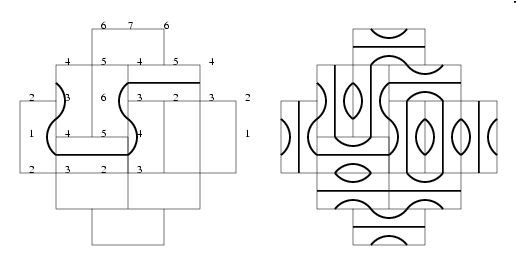If you look at the lines we get versus height functions, you see that we get a contour map. The contour shown represents the contour of height 3.5. There Polynomially many pairings for some reason. We noticed interesting things that go on in the frozen regions.

Nick has been playing with Baxter permutations and helping Abe with the Compatibility Graph Program.

Somebody mentions that there are a lot of mathematicians in France.

Geir forgot to bring the drinks he bought.

Jim gave a beautiful diagonalization proof of that non-trivial theorem he mentioned before.

Then we all went and had a picnic under Van Vleck's tower. That was successful.

# SSL Minutes

15 May 2001
Hal

No one volunteered to bring drinks on Thursday.

Somebody asked Dominic what progress he has made on the Doubled-ASM FPL (The naturally interleaved'' kind). No numbers as yet.

Definition: A pairing is rigid if it has only one corresponding hex-tiling or ASM.

Definition: a pairing is locally rigid if there are no local moves that can be made which preserve the paring but change the tiling/ASM.

If there was a complete set of local moves for a given system, then the two definitions for rigidity would be equivalent.

That reminds me: I was going to look into the question of local moves.

## Fun Summer Projects

Ribbon Tilings

Let me refer you to Chris Moore's email to the domino group.

Date: Wed, 9 May 2001 14:09:40 -0600 (MDT)
From: Cris Moore <moore@santafe.edu>
To: domino nos@pam math.wisc.edu
Subject: ribbon tiles

Fellow domino'ers, you may enjoy finding bijective proofs of the following two things, which I'm thinking of writing a little note about when I get the time. Recall that a ribbon n-omino (or n-ribbon for short) is a path of n squares connected by stepping North or East (note rotations are not allowed). There are 2^(n-1) distinct n-ribbons.

Let N(x,y,n) be the number of tilings of x by y rectangles with n-ribbons. Then

1) for all n > x, N(x,kn,n) is a function only of x and k. For instance, there are exactly as many ways to tile a 3x8 rectangle with 4-ribbons as there are to tile a 3x10 rectangle with 5-ribbons.

2) for all n, N(n,n,n) = n!

Both of these have easy proofs which I'm sure you'll have no trouble finding... just remove diagonals of squares running NW-SE that each ribbon crosses exactly once, converting n-ribbons to (n-1)-ribbons.

- Cris Moore

Jim showed us the easy proof of item (2), then asked us to come up with some other questions. Here they are:

• How many ways to tile a 2n by 2n rectangle with n-ribbons? I came up with a lower bound of (2n)! and thought for a while that that might be an exact answer. But then Jim came up with (n!)^4 as a new lower bound.
• How many ways to tile a 2n by n rectangle with n-ribbons?
• How many ways to tile a l by m rectangle with n-ribbons?
• How many ways to tile a n+1 by n rectangle with n-ribbons?

We then talked about local moves on a ribbon tiling. I forget who invented these. Take two ribbons that lie next to each other for a while and swap their tails. They'll still be the same length, and take up the same space, but be in a different configuration.

Rectangular FPLs

What about square FPLs on a n-by-m grid? You would have to play with the boundary conditions for an odd-by-even grid. Jim said he had dealt with this in part 5 of "The many faces of ASMs". I think that he called them partial ASMs.

## Progress Reports

Nick worked on a project for one of his classes.

Boytcho is writing up some stuff.

Abe studdied up for his exams. This week he'll do the glossary, and over the summer he'll learn about gyration.

Dominic has been obsessing about the sequence 16, 17, 20, 22, 24, 31, 40, 121. He's also been looking at the naturally interleaved'' ASMs, as mentioned before. He wants to modify TOAD Shuffler to show the funny lines mentioned in the notes from two weeks ago.

I have been busy with my paper.

Mike glanced at the eigenvalues of the R-S matrix again.

Jim went to a conference. He talked about Penrose tilings and how to produce one without mistakes by moving a pair of pentagons around the plane. Without benefit of a chalkboard, I didn't follow that very well.

Then we called it quits.

# SSL Minutes

17 May 2001
Dominic & ...

## Baxter Permutations

Hal makes a conjecture that a series of moves such as the one described below can produce (1) only baxter permutations and (2) all of the baxter permutations. He was right on (1), but not on (2). Counterexample for (2): permutation 152436.

Dominic produced the following proof of (1):

Given any identity matrix, we can transpose any any number of 1's along the diagonal. For example, we can take

100000
010000
001000
000100
000010
000001

and transpose the four interior 1's to get
100000
000010
000100
001000
010000
000001


Claim: any such transposition (and successive transpositions) will yield a Baxter Permutation.

Proof: Recall that a Baxter Permutation can be defined as any permutation that fits #.p.p<.p>.p+1.#' where # and #' are unimportant, p is any number in {1,2,...,n-1}, p< is a sequence of numbers less than p, and p> is a sequence of numbers greater than p.

Let [ ] denote the list of numbers that we are transposing. In the previous example, [ ] would contain the numbers 2,3,4,5.

Case 1: Assume p and p+1 are both outside [ ]. This means both are either less than or greater than each number in [ ]. Then all of [ ] is in either # or #'. This is the unimportant stuff. So transposing [ ] does not affect p or p+1. they are still adjacent to each other in the ASM, so p< and p> are both empty. This fits the definition of a Baxter Permutation.

Case 2: Assume p and p+1 are both inside [ ]. Then transposing [ ] will not separate p and p+1. They will still be adjacent to each other. So again, p< and p> are empty. This again fits the definition of a Baxter Permutation.

Case 3: Either p or p+1 is inside [ ], but not both. If p is inside [ ] and p+1 is not, then p must be the maximum element in [ ] (recall we started with the identity matrix). Then transposing [ ], we get: [p-k ... p-2 p-1 p] p+1 -----> [p p-1 p-2 ... p-k] p+1. But this fits the definition of a Baxter permutation with p< containing p-1...p-k and p> as the empty set. If p is outside [ ] and p+1 is inside, then p+1 must be the minimum element in [ ]. Then transposing [ ], we get: p [p+1 p+2 p+3 ... p+k] -----> p [p+k ... p+3 p+2 p+1]. But this fits the definition of a Baxter permutation with p> containing p+1...p+k and p< as the empty set.

Thus, any transposition of elements along the diagonal of an identity matrix (and any successive such transposition) will yield a Baxter Permutation.

That finishes the proof.